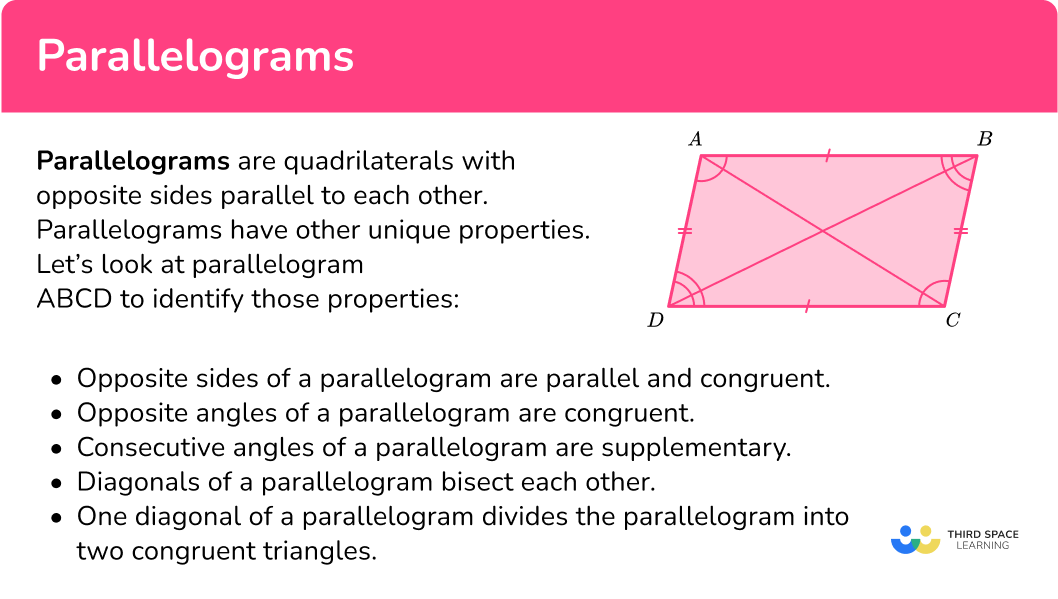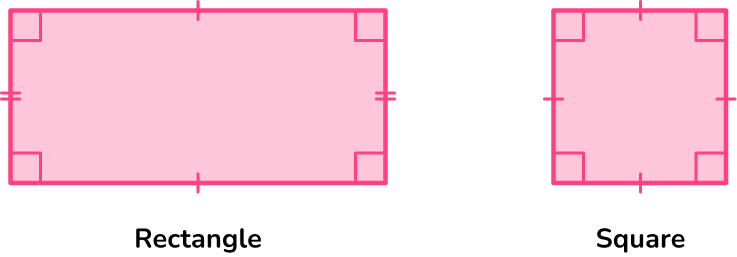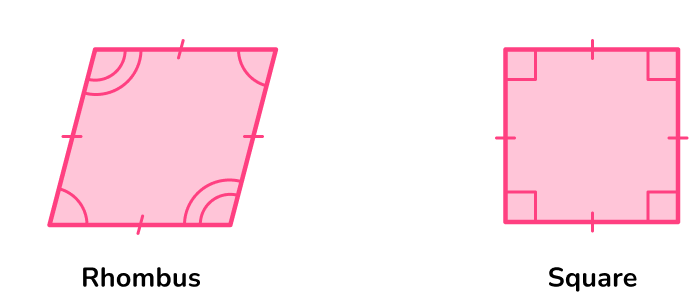# Parallelogram

Here you will learn about parallelograms, including their properties, and special cases.

Students first learn about parallelograms in 3 rd grade with their work in geometry. They expand upon their knowledge of parallelograms as they progress through 5 th grade.

## What are parallelograms?

Parallelograms are quadrilaterals with opposite sides being parallel to each other.

Parallelograms have other unique properties.

### Properties of a Parallelogram

★ Two pairs of opposite congruent sides

• Side AB and Side DC are congruent
• Side AD and Side BC are congruent

★ Two pairs of opposite congruent angles

• Angle A and Angle C are congruent
• Angle D and Angle B are congruent

★ Diagonals bisect each other

• Line segment BD
• Line segment AC

★ Adjacent angles are supplementary meaning they sum to \bf{180^{\circ}}

• angle A + angle B = 180^{\circ}
• angle A + angle D = 180^{\circ}
• angle C + angle B = 180^{\circ}
• angle C + angle D = 180^{\circ}

★ One diagonal divides the parallelogram into two congruent triangles

• Triangle ABC is congruent to triangle CDA
• Triangle DAB is congruent to triangle BCD

Parallelograms also have diagonals. Diagonals are line segments that connect opposite vertices. For example, in the diagram below, line segments AC and BD are diagonals.

There are three types of special parallelograms.

NameShapeAttributes
Rectangle

● Four right angles
● Congruent diagonals

Rhombus

● Four congruent sides
● Perpendicular diagonals

Square

● Four right angles
● Congruent diagonals
● Four congruent sides
● Perpendicular diagonals

To help understand how the parallelograms relate to one another, you can use a Venn diagram.

### What are parallelograms?## Common Core State Standards

How does this relate to 3 rd grade math – 5 th grade math?

• Grade 3 – Geometry (3.G.A.1)
Understand that shapes in different categories (for example, rhombuses, rectangles, and others) may share attributes (like, having four sides), and that the shared attributes can define a larger category (example, quadrilaterals). Recognize rhombuses, rectangles, and squares as examples of quadrilaterals, and draw examples of quadrilaterals that do not belong to any of these subcategories.

• Grade 4 – Geometry (4.G.A.2)
Classify two-dimensional figures based on the presence or absence of parallel or perpendicular lines, or the presence or absence of angles of a specified size. Recognize right triangles as a category, and identify right triangles.

• Grade 5 – Geometry (5.G.3)
Understand that attributes belonging to a category of two-dimensional figures also belong to all subcategories of that category. For example, all rectangles have four right angles and squares are rectangles, so all squares have four right angles.

• Grade 5 – Geometry (5.G.4)
Classify two-dimensional figures in a hierarchy based on properties.

## How to classify parallelograms

In order to classify a parallelogram:

1. Recall the definition and properties of parallelograms.
2. Identify the parallelogram.

## Parallelogram examples

### Example 1: parallelogram

Select the quadrilateral that is a parallelogram.

1. Recall the definition and properties of parallelograms.

Parallelograms are quadrilaterals that have two pairs of opposite parallel sides.

2Identify the parallelogram.

Quadrilateral A is the parallelogram because, by the definition of a parallelogram, there are two pairs of opposite parallel sides.

### Example 2: parallelograms

Kelly drew the quadrilateral below and said it was only a parallelogram. Is she correct?

Parallelograms are quadrilaterals that have opposite parallel sides. The quadrilateral she drew does have opposite parallel sides as well as other properties of parallelograms, such as opposite congruent sides. However, the quadrilateral she drew has four right angles.

The quadrilateral Kelly drew is a special parallelogram. It is a rectangle.

### Example 3: parallelogram

Lucas’s teacher asked him to draw a parallelogram that has four congruent sides but not four congruent angles. Lucas draws a rhombus. Is he correct?

A rhombus is a parallelogram that has 4 equal sides.

Lucas is correct by drawing a rhombus.

### Example 4: square

Name the special parallelogram.

Parallelograms are quadrilaterals that have opposite parallel sides. There are three special parallelograms.

1. A rectangle that has 4 right angles.

2. A rhombus that has 4 equal sides.

3. A square that has 4 right angles and 4 equal sides.

The special parallelogram is a square.

### Example 5: trapezoid

The quadrilateral has one pair of opposite sides that are parallel, therefore is not a parallelogram.

Describe how the quadrilaterals are the same and how they are different. Name them.

Quadrilateral A has two pairs of opposite parallel sides and two pairs of opposite congruent sides. Quadrilateral A also has two pairs of opposite congruent angles.

Quadrilateral B has one pair of opposite parallel sides and two pairs of congruent angles. Quadrilateral B also has one pair of opposite congruent sides.

The quadrilaterals are the same because they have a pair of opposite parallel sides, a pair of opposite congruent sides, and two pairs of congruent angles.

They are different because Quadrilateral A has two pairs of opposite parallel sides and Quadrilateral B only has one pair. Quadrilateral A has two pairs of opposite congruent sides, and quadrilateral B only has one.

Also, Quadrilateral A has two pairs of opposite angles that are congruent, whereas Quadrilateral B has adjacent angles (next to each other) that are congruent.

Quadrilateral B is an isosceles trapezoid.

### Teaching tips for parallelogram

• Have students create a hierarchy of quadrilaterals on their own so that they can investigate the relationships between the sides of a quadrilateral and the angles of a quadrilateral.

• Instead of worksheets, have students engage with geometric digital platforms such as desmos to investigate the properties of quadrilaterals.

• Incorporate project based learning activities which include ways for students to see how the concepts are applied in a real world setting.

### Easy mistakes to make

• Assuming all quadrilaterals with four \bf{90}^{\circ} angles are squares
Rectangles and squares are quadrilaterals with four right angles. If the four sides are equal, then it is a square. If the four sides are not equal, then it is a rectangle.

• Mistaking a trapezoid for a parallelogram
Trapezoids are not classified as parallelograms because they do not have the properties of parallelograms. Trapezoids have only one pair of opposite parallel sides. Parallelograms have two pairs of opposite parallel sides.

• Mistaking a rhombus for a square
A rhombus has four equal sides, but its angles are not right angles like in a square.

### Practice parallelogram questionsrectangletrapezoidsquarerhombusThe quadrilateral has four congruent sides, and the four angles are not congruent. So, the quadrilateral is a rhombus.

2. Which quadrilateral has two pairs of opposite parallel sides and four right angles but does NOT have all sides of equal length?

RhombusRectangleParallelogramSquareQuadrilaterals with two pairs of opposite parallel sides with four right angles have to be a special parallelogram, which is either a rectangle or a square. Since the quadrilateral does not have four congruent sides, it must be a rectangle.3. Which quadrilateral is NOT a parallelogram?The only quadrilateral that cannot be a parallelogram among the choices is the trapezoid.A trapezoid has only one pair of opposite parallel sides, whereas parallelograms have two pairs of opposite parallel sides.

4. Which statement is true?

A square is always a rhombus.A rhombus is always a square.A trapezoid is always a parallelogram.A parallelogram is always a trapezoid.A square is always considered a rhombus because, in order for a quadrilateral to be a rhombus, it has to have 4 congruent sides. Squares have four congruent sides.

However, a rhombus is not always a square because, in order for a quadrilateral to be a square, it has to have four congruent sides and four right angles. Rhombuses do not have four right angles.5. Which quadrilateral could have side lengths 10 \, cm, 6 \, cm, 10 \, cm, 6 \, cm?

RhombusParallelogramTrapezoidSquareParallelograms have two pairs of parallel sides and two pairs of opposite congruent sides. So it is the only quadrilateral choice whose side lengths can be 10 \, cm, 6 \, cm, 10 \, cm, and 6 \, cm.

6. The quadrilateral below can be classified as which of the following?a parallelogram and a rectanglea rectangle and a rhombusa rectangle and a squarea parallelogram and a rhombusA rhombus has two pairs of opposite parallel sides and two pairs of opposite congruent angles which makes it a parallelogram. It also has four congruent sides making it a special type of parallelogram.

## Parallelogram FAQs

What is another name for a quadrilateral?

A quadrilateral is also a four-sided polygon.

What is a trapezium?

A trapezium is another name for a trapezoid.

Do the diagonals of quadrilaterals have special properties?

You will study in detail the diagonals of quadrilaterals in middle and high school. However, they do hold special properties. The diagonals of parallelograms bisect each other. In the special cases of parallelograms, such as rectangles, the diagonals are equal in length, in rhombuses, the diagonals are perpendicular, and in squares, the diagonals are perpendicular bisectors. Kites also have perpendicular diagonals.

How do you find the area of the parallelogram?

The area of a parallelogram is found by using the formula A=b \cdot h

How do you find the perimeter of the parallelogram?

You find the perimeter of a parallelogram the same way you would find the perimeter of any polygon, add up the side lengths.

Can you find the area of a rectangle using the same formula as finding the area of a parallelogram?

Yes, you can use the formula A=b \cdot h to find the area of a rectangle or A=l \cdot w.

What is the sum of the interior angles of a parallelogram?

The sum of the interior angles of a parallelogram is 360^{\circ}.

## Still stuck?

At Third Space Learning, we specialize in helping teachers and school leaders to provide personalized math support for more of their students through high-quality, online one-on-one math tutoring delivered by subject experts.

Each week, our tutors support thousands of students who are at risk of not meeting their grade-level expectations, and help accelerate their progress and boost their confidence.